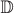Skip to main content

# Table 1 Definition of notations

Notations Definition
N C The number of CUEs per cell
N D The number of DUEs per cellThe set of CUEs in the cellThe set of DUEs in the cell
λ c,n PF metric value of CUE c on subchannel n
λ d,n PF metric value of DUE d on subchannel n
$$G_{{d_{t}} e0}$$ Channel gain between D2D Tx d t and eNB e0
$$\phantom {\dot {i}\!}G_{{d_{t}} {d_{r}}}$$ Channel gain between D2D Tx d t and D2D Rx d r
G c e0 Channel gain between CUE c and eNB e0
$$G_{{c} {d_{r}}}\phantom {\dot {i}\!}$$ Channel gain between CUE c and D2D Rx d r
N 0 Noise power